Professor Murray

Secant and Cosecant Functions

Slide Duration:

Section 1: Trigonometric Functions
Angles

39m 5s

Intro
0:00
Degrees
0:22
Circle is 360 Degrees
0:48
Splitting a Circle
1:13
2:08
2:31
2:52
Half-Circle and Right Angle
4:00
6:24
6:52
Coterminal, Complementary, Supplementary Angles
7:23
Coterminal Angles
7:30
Complementary Angles
9:40
Supplementary Angles
10:08
Example 1: Dividing a Circle
10:38
Example 2: Converting Between Degrees and Radians
11:56
Example 3: Quadrants and Coterminal Angles
14:18
Extra Example 1: Common Angle Conversions
-1
Extra Example 2: Quadrants and Coterminal Angles
-2
Sine and Cosine Functions

43m 16s

Intro
0:00
Sine and Cosine
0:15
Unit Circle
0:22
Coordinates on Unit Circle
1:03
Right Triangles
1:52
2:25
Master Right Triangle Formula: SOHCAHTOA
2:48
Odd Functions, Even Functions
4:40
Example: Odd Function
4:56
Example: Even Function
7:30
Example 1: Sine and Cosine
10:27
Example 2: Graphing Sine and Cosine Functions
14:39
Example 3: Right Triangle
21:40
Example 4: Odd, Even, or Neither
26:01
Extra Example 1: Right Triangle
-1
Extra Example 2: Graphing Sine and Cosine Functions
-2
Sine and Cosine Values of Special Angles

33m 5s

Intro
0:00
45-45-90 Triangle and 30-60-90 Triangle
0:08
45-45-90 Triangle
0:21
30-60-90 Triangle
2:06
Mnemonic: All Students Take Calculus (ASTC)
5:21
Using the Unit Circle
5:59
New Angles
6:21
9:43
Mnemonic: All Students Take Calculus
10:13
13:11
16:48
Example 3: All Angles and Quadrants
20:21
Extra Example 1: Convert, Quadrant, Sine/Cosine
-1
Extra Example 2: All Angles and Quadrants
-2
Modified Sine Waves: Asin(Bx+C)+D and Acos(Bx+C)+D

52m 3s

Intro
0:00
Amplitude and Period of a Sine Wave
0:38
Sine Wave Graph
0:58
Amplitude: Distance from Middle to Peak
1:18
Peak: Distance from Peak to Peak
2:41
Phase Shift and Vertical Shift
4:13
Phase Shift: Distance Shifted Horizontally
4:16
Vertical Shift: Distance Shifted Vertically
6:48
Example 1: Amplitude/Period/Phase and Vertical Shift
8:04
Example 2: Amplitude/Period/Phase and Vertical Shift
17:39
Example 3: Find Sine Wave Given Attributes
25:23
Extra Example 1: Amplitude/Period/Phase and Vertical Shift
-1
Extra Example 2: Find Cosine Wave Given Attributes
-2
Tangent and Cotangent Functions

36m 4s

Intro
0:00
Tangent and Cotangent Definitions
0:21
Tangent Definition
0:25
Cotangent Definition
0:47
Master Formula: SOHCAHTOA
1:01
Mnemonic
1:16
Tangent and Cotangent Values
2:29
Remember Common Values of Sine and Cosine
2:46
90 Degrees Undefined
4:36
Slope and Menmonic: ASTC
5:47
Uses of Tangent
5:54
Example: Tangent of Angle is Slope
6:09
7:49
Example 1: Graph Tangent and Cotangent Functions
10:42
Example 2: Tangent and Cotangent of Angles
16:09
Example 3: Odd, Even, or Neither
18:56
Extra Example 1: Tangent and Cotangent of Angles
-1
Extra Example 2: Tangent and Cotangent of Angles
-2
Secant and Cosecant Functions

27m 18s

Intro
0:00
Secant and Cosecant Definitions
0:17
Secant Definition
0:18
Cosecant Definition
0:33
Example 1: Graph Secant Function
0:48
Example 2: Values of Secant and Cosecant
6:49
Example 3: Odd, Even, or Neither
12:49
Extra Example 1: Graph of Cosecant Function
-1
Extra Example 2: Values of Secant and Cosecant
-2
Inverse Trigonometric Functions

32m 58s

Intro
0:00
Arcsine Function
0:24
Restrictions between -1 and 1
0:43
Arcsine Notation
1:26
Arccosine Function
3:07
Restrictions between -1 and 1
3:36
Cosine Notation
3:53
Arctangent Function
4:30
Between -Pi/2 and Pi/2
4:44
Tangent Notation
5:02
Example 1: Domain/Range/Graph of Arcsine
5:45
Example 2: Arcsin/Arccos/Arctan Values
10:46
Example 3: Domain/Range/Graph of Arctangent
17:14
Extra Example 1: Domain/Range/Graph of Arccosine
-1
Extra Example 2: Arcsin/Arccos/Arctan Values
-2
Computations of Inverse Trigonometric Functions

31m 8s

Intro
0:00
Inverse Trigonometric Function Domains and Ranges
0:31
Arcsine
0:41
Arccosine
1:14
Arctangent
1:41
Example 1: Arcsines of Common Values
2:44
Example 2: Odd, Even, or Neither
5:57
Example 3: Arccosines of Common Values
12:24
Extra Example 1: Arctangents of Common Values
-1
Extra Example 2: Arcsin/Arccos/Arctan Values
-2
Section 2: Trigonometric Identities
Pythagorean Identity

19m 11s

Intro
0:00
Pythagorean Identity
0:17
Pythagorean Triangle
0:27
Pythagorean Identity
0:45
Example 1: Use Pythagorean Theorem to Prove Pythagorean Identity
1:14
Example 2: Find Angle Given Cosine and Quadrant
4:18
Example 3: Verify Trigonometric Identity
8:00
Extra Example 1: Use Pythagorean Identity to Prove Pythagorean Theorem
-1
Extra Example 2: Find Angle Given Cosine and Quadrant
-2
Identity Tan(squared)x+1=Sec(squared)x

23m 16s

Intro
0:00
Main Formulas
0:19
Companion to Pythagorean Identity
0:27
For Cotangents and Cosecants
0:52
How to Remember
0:58
Example 1: Prove the Identity
1:40
Example 2: Given Tan Find Sec
3:42
Example 3: Prove the Identity
7:45
Extra Example 1: Prove the Identity
-1
Extra Example 2: Given Sec Find Tan
-2

52m 52s

Intro
0:00
0:09
How to Remember
0:48
Cofunction Identities
1:31
How to Remember Graphically
1:44
Where to Use Cofunction Identities
2:52
Example 1: Derive the Formula for cos(A-B)
3:08
Example 2: Use Addition and Subtraction Formulas
16:03
Example 3: Use Addition and Subtraction Formulas to Prove Identity
25:11
Extra Example 1: Use cos(A-B) and Cofunction Identities
-1
Extra Example 2: Convert to Radians and use Formulas
-2
Double Angle Formulas

29m 5s

Intro
0:00
Main Formula
0:07
How to Remember from Addition Formula
0:18
Two Other Forms
1:35
Example 1: Find Sine and Cosine of Angle using Double Angle
3:16
Example 2: Prove Trigonometric Identity using Double Angle
9:37
Example 3: Use Addition and Subtraction Formulas
12:38
Extra Example 1: Find Sine and Cosine of Angle using Double Angle
-1
Extra Example 2: Prove Trigonometric Identity using Double Angle
-2
Half-Angle Formulas

43m 55s

Intro
0:00
Main Formulas
0:09
Confusing Part
0:34
Example 1: Find Sine and Cosine of Angle using Half-Angle
0:54
Example 2: Prove Trigonometric Identity using Half-Angle
11:51
Example 3: Prove the Half-Angle Formula for Tangents
18:39
Extra Example 1: Find Sine and Cosine of Angle using Half-Angle
-1
Extra Example 2: Prove Trigonometric Identity using Half-Angle
-2
Section 3: Applications of Trigonometry
Trigonometry in Right Angles

25m 43s

Intro
0:00
Master Formula for Right Angles
0:11
SOHCAHTOA
0:15
Only for Right Triangles
1:26
Example 1: Find All Angles in a Triangle
2:19
Example 2: Find Lengths of All Sides of Triangle
7:39
Example 3: Find All Angles in a Triangle
11:00
Extra Example 1: Find All Angles in a Triangle
-1
Extra Example 2: Find Lengths of All Sides of Triangle
-2
Law of Sines

56m 40s

Intro
0:00
Law of Sines Formula
0:18
SOHCAHTOA
0:27
Any Triangle
0:59
Graphical Representation
1:25
Solving Triangle Completely
2:37
When to Use Law of Sines
2:55
ASA, SAA, SSA, AAA
2:59
SAS, SSS for Law of Cosines
7:11
Example 1: How Many Triangles Satisfy Conditions, Solve Completely
8:44
Example 2: How Many Triangles Satisfy Conditions, Solve Completely
15:30
Example 3: How Many Triangles Satisfy Conditions, Solve Completely
28:32
Extra Example 1: How Many Triangles Satisfy Conditions, Solve Completely
-1
Extra Example 2: How Many Triangles Satisfy Conditions, Solve Completely
-2
Law of Cosines

49m 5s

Intro
0:00
Law of Cosines Formula
0:23
Graphical Representation
0:34
Relates Sides to Angles
1:00
Any Triangle
1:20
Generalization of Pythagorean Theorem
1:32
When to Use Law of Cosines
2:26
SAS, SSS
2:30
Heron's Formula
4:49
Semiperimeter S
5:11
Example 1: How Many Triangles Satisfy Conditions, Solve Completely
5:53
Example 2: How Many Triangles Satisfy Conditions, Solve Completely
15:19
Example 3: Find Area of a Triangle Given All Side Lengths
26:33
Extra Example 1: How Many Triangles Satisfy Conditions, Solve Completely
-1
Extra Example 2: Length of Third Side and Area of Triangle
-2
Finding the Area of a Triangle

27m 37s

Intro
0:00
Master Right Triangle Formula and Law of Cosines
0:19
SOHCAHTOA
0:27
Law of Cosines
1:23
Heron's Formula
2:22
Semiperimeter S
2:37
Example 1: Area of Triangle with Two Sides and One Angle
3:12
Example 2: Area of Triangle with Three Sides
6:11
Example 3: Area of Triangle with Three Sides, No Heron's Formula
8:50
Extra Example 1: Area of Triangle with Two Sides and One Angle
-1
Extra Example 2: Area of Triangle with Two Sides and One Angle
-2
Word Problems and Applications of Trigonometry

34m 25s

Intro
0:00
Formulas to Remember
0:11
SOHCAHTOA
0:15
Law of Sines
0:55
Law of Cosines
1:48
Heron's Formula
2:46
Example 1: Telephone Pole Height
4:01
Example 2: Bridge Length
7:48
Example 3: Area of Triangular Field
14:20
Extra Example 1: Kite Height
-1
Extra Example 2: Roads to a Town
-2
Vectors

46m 42s

Intro
0:00
Vector Formulas and Concepts
0:12
Vectors as Arrows
0:28
Magnitude
0:38
Direction
0:50
Drawing Vectors
1:16
Uses of Vectors: Velocity, Force
1:37
Vector Magnitude Formula
3:15
Vector Direction Formula
3:28
Vector Components
6:27
Example 1: Magnitude and Direction of Vector
8:00
Example 2: Force to a Box on a Ramp
12:25
Example 3: Plane with Wind
18:30
Extra Example 1: Components of a Vector
-1
Extra Example 2: Ship with a Current
-2
Section 4: Complex Numbers and Polar Coordinates
Polar Coordinates

1h 7m 35s

Intro
0:00
Polar Coordinates vs Rectangular/Cartesian Coordinates
0:12
Rectangular Coordinates, Cartesian Coordinates
0:23
Polar Coordinates
0:59
Converting Between Polar and Rectangular Coordinates
2:06
R
2:16
Theta
2:48
Example 1: Convert Rectangular to Polar Coordinates
6:53
Example 2: Convert Polar to Rectangular Coordinates
17:28
Example 3: Graph the Polar Equation
28:00
Extra Example 1: Convert Polar to Rectangular Coordinates
-1
Extra Example 2: Graph the Polar Equation
-2
Complex Numbers

35m 59s

Intro
0:00
Main Definition
0:07
Number i
0:23
Complex Number Form
0:33
Powers of Imaginary Number i
1:00
Repeating Pattern
1:43
Operations on Complex Numbers
3:30
3:39
Multiplying Complex Numbers
4:39
FOIL Method
5:06
Conjugation
6:29
Dividing Complex Numbers
7:34
Conjugate of Denominator
7:45
Example 1: Solve For Complex Number z
11:02
Example 2: Expand and Simplify
15:34
Example 3: Simplify the Powers of i
17:50
Extra Example 1: Simplify
-1
Extra Example 2: All Complex Numbers Satisfying Equation
-2
Polar Form of Complex Numbers

40m 43s

Intro
0:00
Polar Coordinates
0:49
Rectangular Form
0:52
Polar Form
1:25
R and Theta
1:51
Polar Form Conversion
2:27
R and Theta
2:35
Optimal Values
4:05
Euler's Formula
4:25
Multiplying Two Complex Numbers in Polar Form
6:10
Multiply r's Together and Add Exponents
6:32
Example 1: Convert Rectangular to Polar Form
7:17
Example 2: Convert Polar to Rectangular Form
13:49
Example 3: Multiply Two Complex Numbers
17:28
Extra Example 1: Convert Between Rectangular and Polar Forms
-1
Extra Example 2: Simplify Expression to Polar Form
-2
DeMoivre's Theorem

57m 37s

Intro
0:00
Introduction to DeMoivre's Theorem
0:10
n nth Roots
3:06
DeMoivre's Theorem: Finding nth Roots
3:52
Relation to Unit Circle
6:29
One nth Root for Each Value of k
7:11
Example 1: Convert to Polar Form and Use DeMoivre's Theorem
8:24
Example 2: Find Complex Eighth Roots
15:27
Example 3: Find Complex Roots
27:49
Extra Example 1: Convert to Polar Form and Use DeMoivre's Theorem
-1
Extra Example 2: Find Complex Fourth Roots
-2
Bookmark & Share Embed

## Copy & Paste this embed code into your website’s HTML

Please ensure that your website editor is in text mode when you paste the code.
(In Wordpress, the mode button is on the top right corner.)
×
• - Allow users to view the embedded video in full-size.
Since this lesson is not free, only the preview will appear on your website.

• ## Related Books1 answerLast reply by: Dr. William MurrayWed Jul 1, 2015 9:02 AMPost by Brandon Dorman on June 30, 2015Hello,I am trying to wrap my head around this concept. I understand sec=1/cos. but I ran into a problem that has me confused.The question was:What is the same as sec(2Ï€/3)?a. sec(-Ï€/3b. sec(-5Ï€/3c. -sec(5Ï€/3)d. -sec(Ï€/3)The answer is d. but I'm completely confuse as why. Thank you!3 answersLast reply by: Dr. William MurraySat Jul 5, 2014 6:10 PMPost by Austin Cunningham on June 11, 2014I have only taken Algebra 1 and Geometry, so I am sometimes utterly confused when you use words I am vaguely familiar with like "asymptotes" and "local minimums" and "local maximums"? Would you mind explaining what exactly the types of max(es) and minimums there are?1 answerLast reply by: Dr. William MurrayTue May 13, 2014 4:46 PMPost by Kenneth Montfort on May 9, 2014Could you tell me what lecture stated where sine is an odd function and cosine is an even function?1 answerLast reply by: Dr. William MurrayThu Apr 18, 2013 12:15 PMPost by abbas esmailzadeh on May 22, 2012i dont undrstnad if the secant function repeat at 3pi/2 or at2 pi.i am talking about the period.1 answerLast reply by: Dr. William MurrayThu Apr 18, 2013 12:10 PMPost by Yvonne Hamnquist on February 20, 2012A tourist wants to determine the height of the Eiffel Tower without looking in her guide book. She observes the angle of the elevation of the top of the tower from one point on the street is 31.73 degrees. She moves 200m closer to the tower and observes an angle of elevation to the top of the tower of 45 degrees. What is the height of the tower to the nearest meter?Can anyone help?1 answerLast reply by: Dr. William MurrayThu Apr 18, 2013 11:54 AMPost by David Burns on August 17, 2011The "Quick Notes" section here does not correspond to the lecture. There are a couple other cases on this course where this happens.

### Secant and Cosecant Functions

Main definitions:

• The secant function is defined by
 secθ = 1 cosθ
for values of θ where cosθ≠ 0. (For values of θ where cosθ = 0, the secant is undefined.)
• The cosecant function is defined by
 cscθ = 1 sinθ
for values of θ where sinθ≠ 0. (For values of θ where sinθ = 0, the cosecant is undefined.)

Example 1:

Draw a graph of the secant function. Label all zeroes, maxes, mins, and asymptotes. What is the period of the secant function?

Example 2:

Find the values of secant and cosecant at the common angles in the first quadrant: 0, (π/6), (π /4),)(π/3), (π/2). Determine in which other quadrants the secant and cosecant are positive.

Example 3:

Determine if the secant and cosecant functions are odd, even, or neither.

Example 4:

Draw a graph of the cosecant function. Label all zeroes, maxes, mins, and asymptotes. What is the period of the cosecant function?

Example 5:

Find the secants and cosecants of (5π/6)R, 240° , and (7π/4)R.

### Secant and Cosecant Functions

Find the secant and the cosecant of 120°
• Find the sine and cosine of 120°. Note that 120° = [(2π)/3]
• Locate 120° on the unit circle.
•• We know that cosine is the x coordinate of 120° and sine is the y coordinate of 120°
• sin120° = [(√3 )/2], and cos120° = − [1/2]
• cscθ = [1/(sinθ)], and secθ = [1/(cosθ)]
csc120° = [1/([(√3 )/2])] = [(2√3 )/3], sec120° = [1/( − [1/2])] = − 2
Find the secant and the cosecant of [(11π)/6]
• Find the sine and cosine of [(11π)/6]. Note that [(11π)/6] = 330°
• Locate [(11π)/6] on the unit circle.
•• We know that cosine is the x coordinate of [(11π)/6] and sine is the y coordinate of [(11π)/6]
• sin[(11π)/6] = − [1/2], and cos[(11π)/6] = [(√3 )/2]
• cscθ = [1/(sinθ)], and secθ = [1/(cosθ)]
csc[(11π)/6] = [1/( − [1/2])] = − 2 , sec[(11π)/6] = [1/([(√3 )/2])] = [(2√3 )/3]
Find the secant and the cosecant of 225°
• Find the sine and cosine of 225°. Note that 225° = [(5π)/4]
• Locate 225° on the unit circle.
•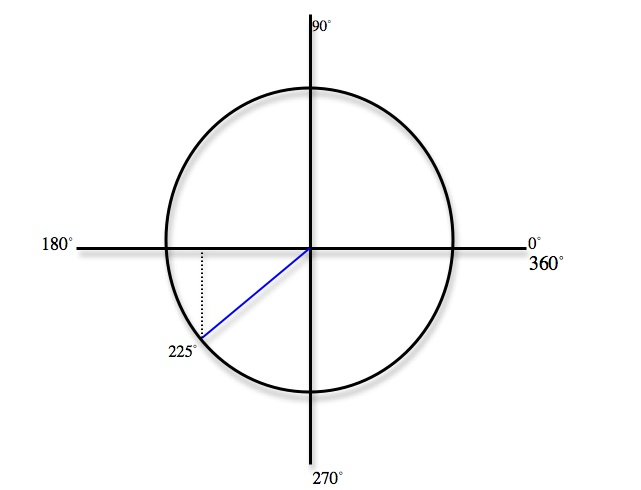• We know that cosine is the x coordinate of 225° and sine is the y coordinate of 225°
• sin225° = − [(√2 )/2], and cos225° = − [(√2 )/2]
• cscθ = [1/(sinθ)], and secθ = [1/(cosθ)]
csc225° = − √2, sec225° = − √2
Find the secant and the cosecant of [(3π)/4]
• Find the sine and cosine of [(3π)/4]. Note that [(3π)/4] = 135°
• Locate [(3π)/4] on the unit circle.
•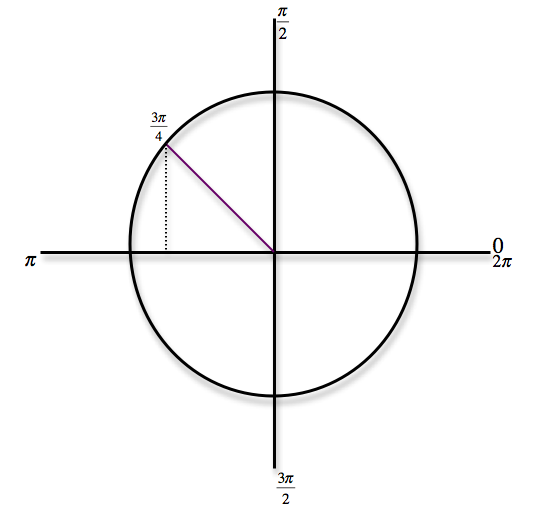• We know that cosine is the x coordinate of [(3π)/4] and sine is the y coordinate of [(3π)/4]
• sin[(3π)/4] = [(√2 )/2], and cos[(3π)/4] = − [(√2 )/2]
• cscθ = [1/(sinθ)], and secθ = [1/(cosθ)]
csc[(3π)/4] = √2, sec[(3π)/4] = − √2
Find the secant and the cosecant of 300°
• Find the sine and cosine of 300°. Note that 300° = [(5π)/3]
• Locate 300° on the unit circle.
•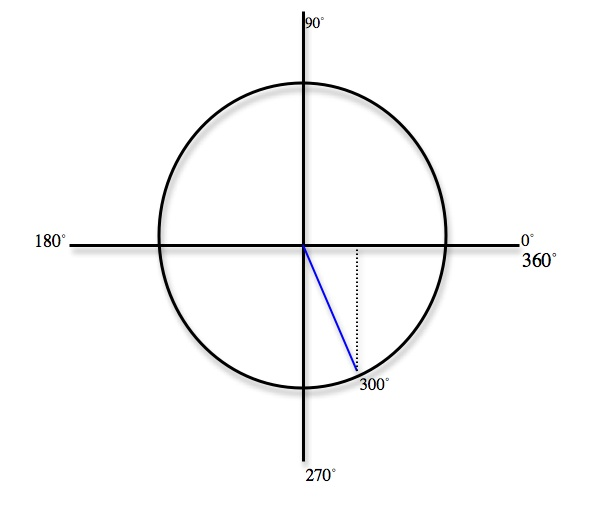• We know that cosine is the x coordinate of 300° and sine is the y coordinate of 300°
• sin300° = − [(√3 )/2], and cos300° = [1/2]
• cscθ = [1/(sinθ)], and secθ = [1/(cosθ)]
csc300° = − [(2√3 )/3], sec300° = 2
Find the secant and the cosecant of [(7π)/6]
• Find the sine and cosine of [(7π)/6]. Note that [(7π)/6] = 210°
• Locate [(7π)/6] on the unit circle.
•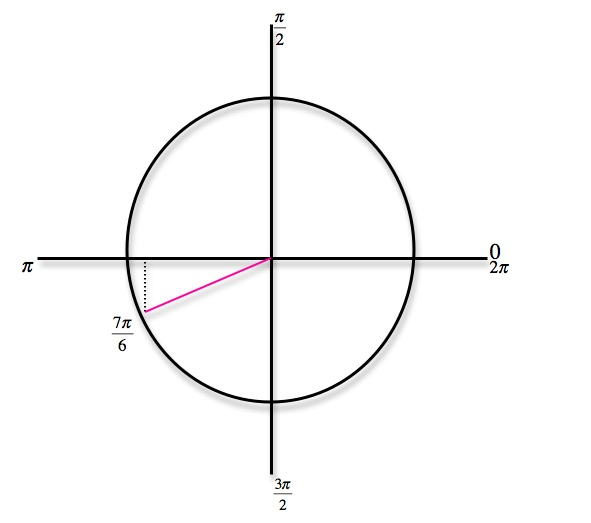• We know that cosine is the x coordinate of [(7π)/6] and sine is the y coordinate of [(7π)/6]
• sin[(7π)/6] = − [1/2], and cos[(7π)/6] = − [(√3 )/2]
• cscθ = [1/(sinθ)], and secθ = [1/(cosθ)]
csc[(7π)/6] = − 2, sec[(7π)/6] = − [(2√3 )/3]
Graph the function f(x) = sec[x/2]. Identify the zeroes, maxima, minima, asymptotes, and the period.
• Start by graphing f(x) = cos[x/2]. Calculate the period by solving [x/2] = 0 and [x/2] = 2π. x = 0 and x = 4π
•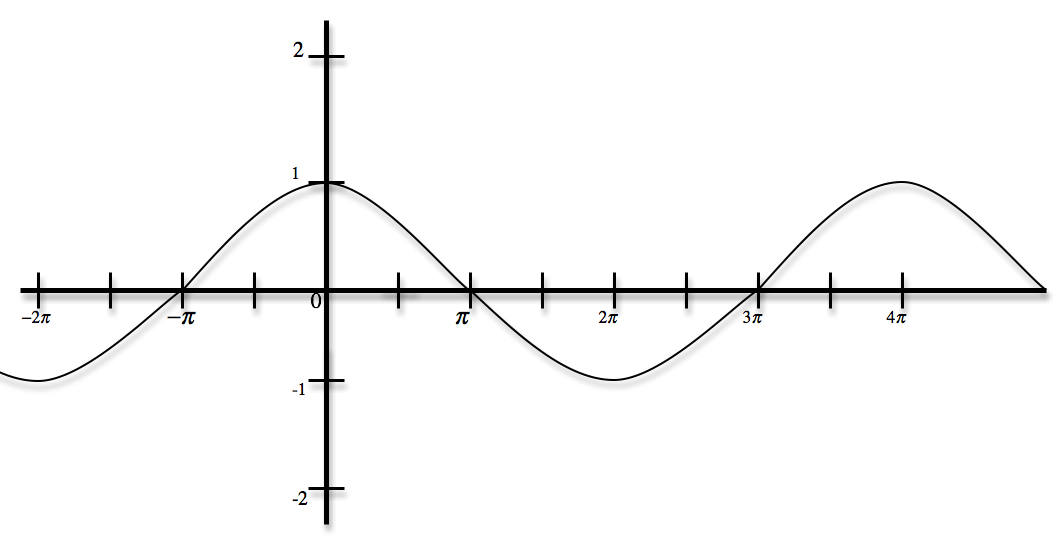• The zeroes of f(x) = cos[x/2] are the vertical asymptotes of f(x) = sec[x/2]. Now graph f(x) = sec[x/2].
•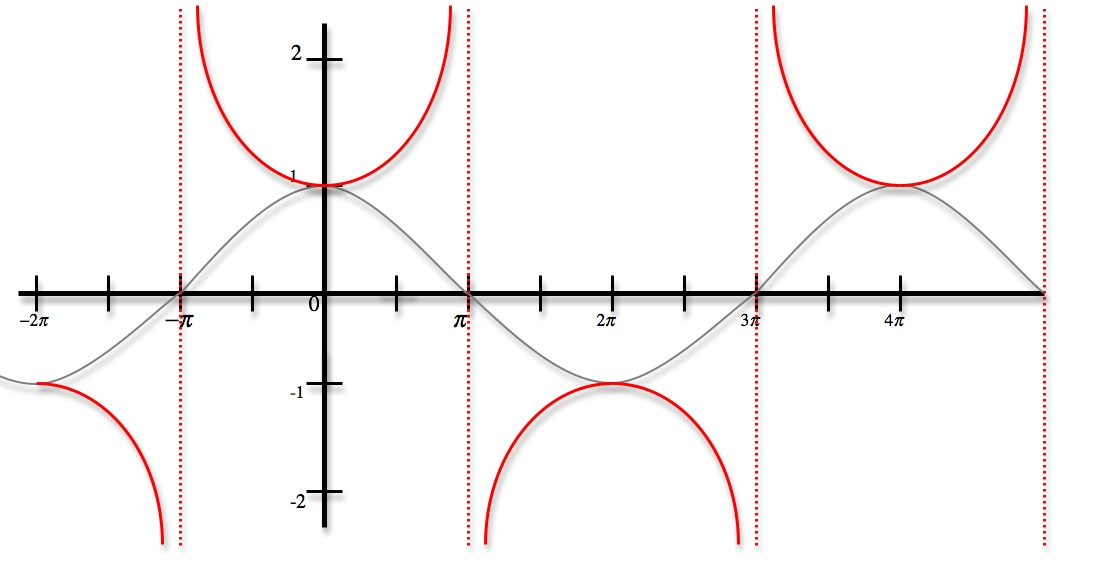Zeroes: has no zeroes, Maxima: ( − 2π, − 1), (2π, − 1), Minima: (0, 1), (4π, 1), Asymptotes: − π, π, 3π, Period: 4π
Graph the function f(x) = csc2x. Identify the zeroes, maxima, minima, asymptotes, and the period.
• Start by graphing f(x) = sin2x. Calculate the period by solving 2x = 0 and 2x = 2π. x = 0 and x = π
•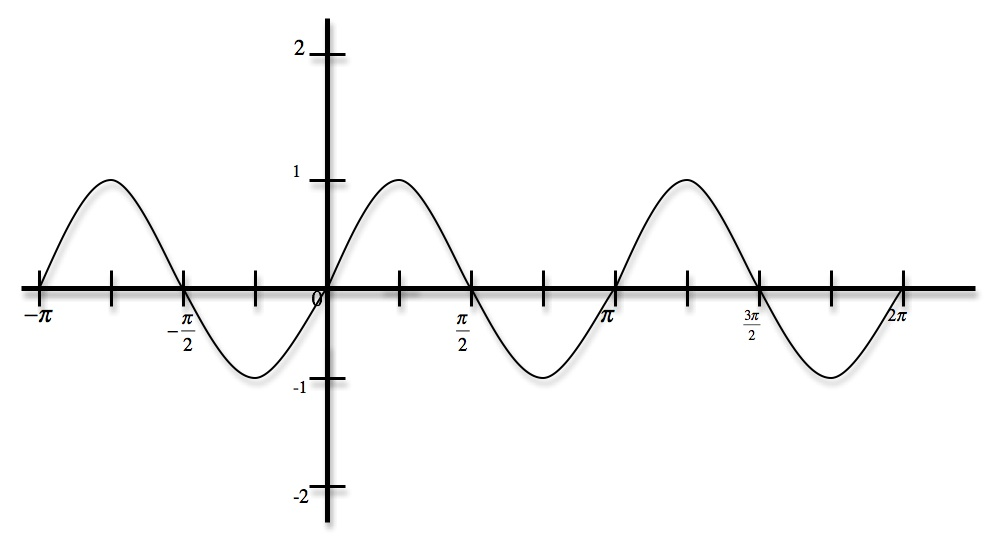• The zeroes of f(x) = sin2x are the vertical asymptotes of f(x) = csc2x. Now graph f(x) = csc2x.
•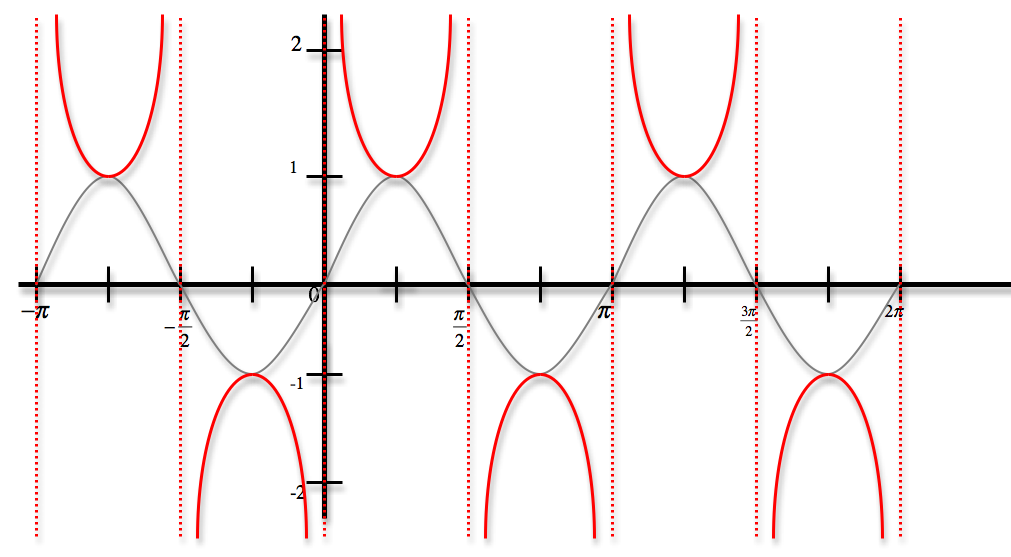Zeroes: has no zeroes, Maxima: ( − [(π)/4], − 1), ([(3π)/4], − 1), ([(7π)/4], − 1), Minima: ([(π)/4], 1), ([(5π)/4], 1), Asymptotes: − [(π)/2], 0, [(π)/2], π, [(3π)/2], 2π, Period: π
Graph the function f(x) = - 2sec2x. Identify the zeroes, maxima, minima, asymptotes, and the period.
• Start by graphing f(x) = - 2cos2x. Calculate the period by solving 2x = 0 and 2x = 2π. x = 0 and x = π
•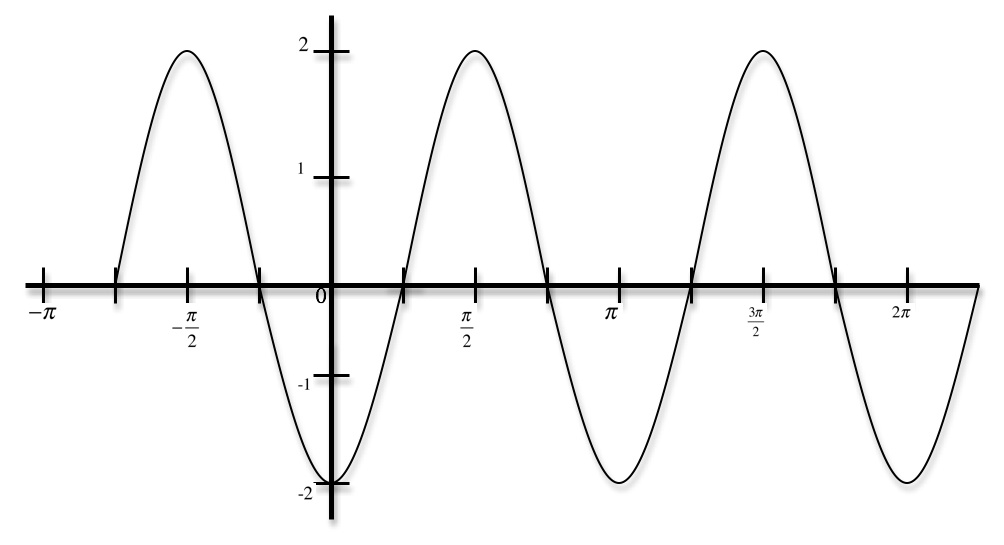• The zeroes of f(x) = - 2cos2x are the vertical asymptotes of f(x) = - 2sec2x. Now graph f(x) = - 2sec2x.
•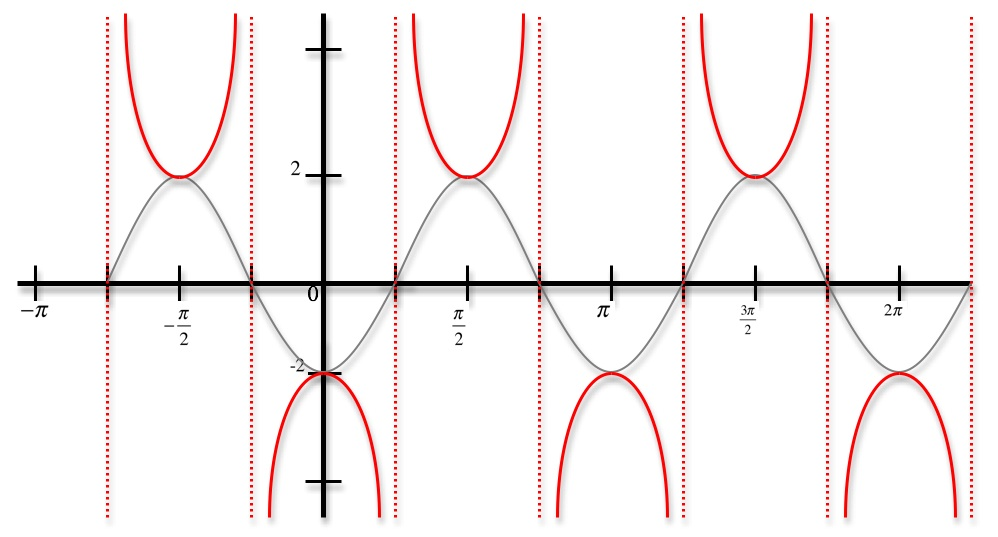Zeroes: has no zeroes, Maxima: (0, − 2), (π, − 2), (2π, − 2), Minima: ([(π)/2], 2), ([(3π)/2], 2), Asymptotes: − [(π)/4], [(π)/4], [(3π)/4], [(5π)/4], Period: π
Graph the function f(x) = − [1/2]csc[x/2]. Identify the zeroes, maxima, minima, asymptotes, and the period.
• Start by graphing f(x) = − [1/2]sin[x/2]. Calculate the period by solving [x/2] = 0 and [x/2] = 2π. x = 0 and x = 4π
•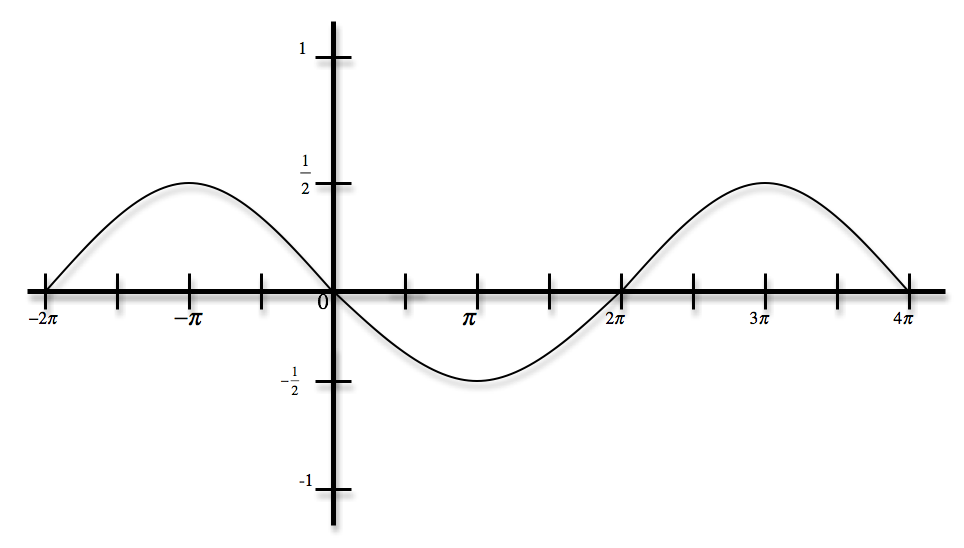• The zeroes of f(x) = − [1/2]sin[x/2] are the vertical asymptotes of f(x) = − [1/2]csc[x/2]. Now graph f(x) = − [1/2]csc[x/2].
•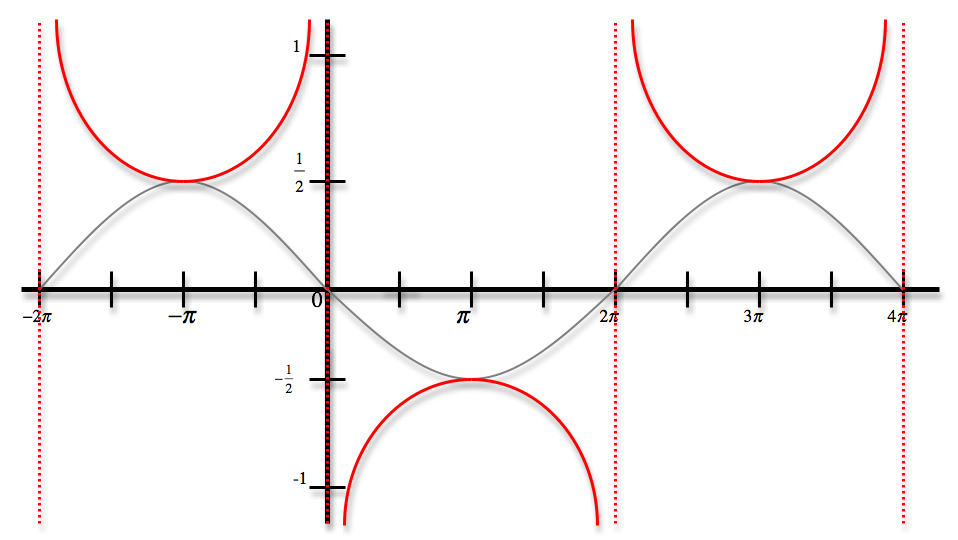Zeroes: has no zeroes, Maxima: (π, − [1/2]), (5π, − [1/2]), Minima: ( − π, [1/2]), (3π, [1/2]), Asymptotes: − 2π, 0, 2π, 4π, Period: 4π

*These practice questions are only helpful when you work on them offline on a piece of paper and then use the solution steps function to check your answer.

### Secant and Cosecant Functions

Lecture Slides are screen-captured images of important points in the lecture. Students can download and print out these lecture slide images to do practice problems as well as take notes while watching the lecture.

• Intro 0:00
• Secant and Cosecant Definitions 0:17
• Secant Definition
• Cosecant Definition
• Example 1: Graph Secant Function 0:48
• Example 2: Values of Secant and Cosecant 6:49
• Example 3: Odd, Even, or Neither 12:49
• Extra Example 1: Graph of Cosecant Function
• Extra Example 2: Values of Secant and Cosecant

### Transcription: Secant and Cosecant Functions

We are trying some more examples for the sec and cosec function.0000

Here we are being asked to draw a graph of the cosec function and we have to label all 0, max, min, and asymptotes, and identify the period.0005

The key thing to remember here is the cosec(theta) is 1/sin(theta), really this comes down to understanding the graph sin(theta) very well.0015

I’m going to start with a graph of sin(theta), that is probably a graph that you should have already memorized.0029

My graph of sin(theta) goes up to 1 and down to -1, starts at 0 goes up to 1 and pi/2, down to 0 at pi, down to -1 that is 3pi/2 and back at 0 at 2pi.0048

What I have drawn there is not cosec yet, it is sin(theta), in red I will draw cosec(theta).0073

Cosec(theta) is just 1/sin(theta), whenever sin is 1 it is 1, whenever sin is 1- it is -1, whenever sin is 0 where you can not divide by 0, that is where cosec has an asymptote.0083

It goes up to infinity whenever sin goes down to 0.0110

And down to negative infinity whenever sin goes down to 0.0119

It looks a lot like the graph of sec did, it got these U’s going up to infinity and upside down U’s going down to negative infinity depending on where the graph of sin hits 0.0127

Let us label everything we have been asked to label here, cosec(theta) never crosses the (x) axis, so it has no 0.0143

There are no 0 to label there, max and min it has local min at the bottom of each of these U’s.0158

This is at pi/2 and 1, that is a local min.0165

We have local max at –pi/2 and -1, that is a local max, and another one at 3pi/2, -1, that is our local max.0174

We got the max and min, the asymptotes are places where it goes up to infinity and down to negative infinity.0197

Let me label those, there is one right there at –pi.0205

Here is another one at 0, another one at pi, and finally here is one at 2pi.0215

Basically those are the places where sin(theta) is 0, remember cosec is 1/sin(theta) so whenever you are trying to divide by 0, that is where (cosec) blows up to infinity or drops down to negative infinity.0228

We got the asymptotes, the period of the (cosec) function is how long it takes to repeat itself.0242

That is one period before it starts repeating itself and that is 2pi and that really comes back to the fact that sin(theta) has a period of 2 pi.0253

The period of (cosec) just like (sec) is 2 pi.0262

It looks like we answered everything there, the key part there is remember that (cosec) is 1/sin, you probably do not need to memorize the graph of (cosec) itself.0273

It is good if you are familiar with this general shape of the U’s going up and the U’s going down but you do not need to memorize the details.0285

As long as you remember that it is 1/sin, you can work it out from there.0295

Ok for our next example here, we are given a bunch of angles and we have to find the (sec) and (cosec) of these common values.0000

Let me start by drawing these angles on a unit circle, that is a squish unit circle, let me see if I can do a little better there.0009

This is 0, pi/2, pi/, 3pi/2, and 2pi, it looks like at least one of these angles is given in degrees as well.0036

I will label the values in degrees, there is 90, 180, 270, and 360, those are the values in degrees.0049

Let us figure out where these angles are in the unit circle.0060

5pi/6 is over here, 240 degrees is between 180 and 270, that is down here.0063

7pi/4 is between 3pi/2 and 2pi, that is down there.0075

Remember (sec) and (cosec), those are that reciprocals of (cos) and (sin).0082

The smart thing to do here is to figure out the (cos) and (sin) of those angles and then it would be an easy matter to find the (sec) and (cosec).0087

I’m going to make a little chart, the angle, (cos), (sin) and then it would be an easy matter to find the (sec) and (cosec).0099

Let us start with 5pi/6, that is over here and that is a 30, 60, 90 triangle so we know the values.0115

The (cos) is (root 3)/2, (sin) is ½, but then we have to worry about whether they are positive or negative.0123

The (x) coordinate is negative so I will make the (cos) negative.0130

The (sec) now is just 1/cos, so that is 2/square root 3, but if we rationalized that, that is 2 root 3/3 and of course it is negative.0135

The (cosec) is 1/sin so 1/(1/2) is just 2.0148

Now the next one is 240 degrees, if you like to translate into radians, that is 4pi/3, that is this angle down here.0156

We see a 30, 60, 90 triangle, this time the (cos) is the short side that is ½, the (sin) is the long side root 3/2.0176

But it is in the third quadrant so they are both negative.0187

The (sec) here is 1/cos, so that is -2, (cosec) 1/sin, 2/root 3 rationalizes to (2 root 3)/3 and that is negative.0192

Finally, 7pi/4 that is this angle over here.0207

It helps to start out with the (cos) and (sin), that is a 45 degrees triangle so we know they are both root 2/2.0219

We just have to figure out which one is positive and which one is negative.0226

The (y) coordinate is negative there so the (sin) must be negative.0230

The (sec) is 1/(cos), 2 /root 2 is just root 2, (cosec)=1/(sin) is 2/root 2 so that is root 2 again but it is negative.0235

I really emphasize to my students that you do not really need to memorize the (sec) and (cosec) of the common values if you really have the (sin) and (cos) memorized well.0250

I think it is more important to memorize the (sin) and (cos) very well and the way you get those is by knowing those common values for the 30, 60, 90 triangles and for the 45, 45, 90 triangles.0264

You use those to figure out the (sin) and (cos), you figure out which quadrant you are in, which tells you whether they are positive or negative.0278

Now you know (sin) and (cos), whether they are positive or negative.0287

For (sec) and (cosec), all you have to remember is that sec(theta)=1/cos(theta).0291

If you can figure out the (cos), you can figure out (sec).0300

Similarly for the cosec(theta) is 1/sin(theta), again if you figure out the sin(theta) it is a simple matter to figure out the cosec(theta).0303

I hope all those worked out well for you, this is part of the trigonometry lectures series for www.educator.com.0315

Hi welcome back to the trigonometry lectures on educator.com0000

Today, we're going to learn about the last trigonometric functions.0004

We've learned about the sine and cosine, and tangent and cotangent.0008

Today we're going to learn the secant and cosecant function.0011

The secant function is just defined by the sec(θ)=1/cos(θ).0019

That only works when the cos(θ) is non-zero.0025

If the (cosθ)=0, we just say the secant is undefined.0027

The cosecant, which people shorten to csc, is just 1/sin(θ).0033

If the sin(θ)=0, we just say that the cosecant is undefined.0040

We have to draw a graph of the secant function.0051

In particular, we have to label all zeros, max's, mins, and asymptotes and figure what the period of the secant function is.0055

Remember now that the secant function, sec(θ), by definition is 1/cos(θ).0064

A really good place to start here when you're trying to understand sec(θ) is with the graph of cos(θ).0073

There's π, there's 2π, and I'm going to extend this out a bit, 3π, and -π.0086

Now, remember that the cosine function starts at 1, goes down to 0 at π/2, so there's π/2, goes down to -1 at π, comes back to 0 at 3π/2, back up to 1 at 2π.0098

The period of cosine is 2π, so it's repeating itself after 2π.0130

I'm not drawing secant yet, I'm drawing cos(x), y=cos(x).0140

In black here, I've got, we'll call it cos(θ).0146

Now, I'm going to draw the secant function in red.0153

That means we're doing 1/cos(x), or 1/cos(θ).0159

In particular, 1/1 is 1.0165

Then as the cosine goes down to 0, secant is 1/cos, so it goes up to infinity there, and does the same thing on the other side, so secant looks like that.0170

When the cosine is negative, when the cosine is -1, secant is -1.0201

When the cosine goes to 0, secant blows up but since cosine is negative here, secant goes to negative infinity when the cosine is negative.0217

Then when cosine is positive again, secant is positive again, going up to positive infinity on both ends there.0235

That's what the secant function looks like.0247

While the cosine goes between -1 and 1, secant is just the reciprocal of that, so it goes 1 up to negative infinity, and from -1 down to negative infinity.0249

Now, I've got the secant graph in red.0260

Let me label all the things that we've been asked to label.0267

First of all, zeros, while the sec(θ) has no zeros because it never crosses the x-axis, so there are no zeros to label.0270

Max's, all local max's of the secant function, well here's one down here at (π,-1) is a local max.0282

Minimum at (0,1) is a local min, (2π,1) is a local min, and so on, as a local min and a local max, every π units.0295

We've got the max's and the mins.0314

The asymptotes are the places where the secant blows up to infinity or drops down to negative infinity and so that's an asymptote at -π/2, and at π/2.0318

We have an asymptote at π/2, and again at 3π/2, and again at 5π/2.0333

Basically, every π units, we have an asymptote where the secant function blows up to infinity or drops down to negative infinity.0350

Finally, what is the period of secant function.0362

The secant function is really dependent on the cosine function, and the cosine function repeats itself once every 2π.0367

The period of cosine is 2π.0374

The period of secant is also 2π.0376

You can see that from the graph, it starts repeating itself after a multiple of 2π.0384

That's what the graph of the secant function looks like.0394

It kind of has this hues and this upside down hues really dependent on the cosine function because the secant is just 1/cos(θ).0399

For the second example, we have to figure out some common values of secant and cosecant at the angles in the first quadrant, 0, π/6, π/4, π/3, and π/2.0410

Now, these angles are probably so common that you really should have memorized the sine and cosine.0426

I'm going to start by writing down the sine and cosine of these values.0433

I'm going to write them down both in degrees and radians because it's very important to be able to identify these common values either way.0440

I'll write down degrees, radians, I'll write down the cosine and the sine, and then the secant and cosecant of each one.0449

I'll make a nice chart here.0465

The values given were 0, π/6, π/4, π/3, and π/2, in terms of degrees, that's 0, 30, 45, 60, and 90.0467

Now, you really should have probably memorized the cosine and sine of these already.0486

You probably shouldn't even have to check the unit circle.0493

But if you need to, go ahead and draw yourself a unit circle.0496

Then draw out those triangles in the first quadrant, and you'll be able to figure out the cosine and sine very quickly as long as you remember the values of the 30-60-90 triangles and the 45-45-90 triangles.0500

In particular, the cosine and sine of 0, are 1 and 0.0510

For 30-degree angle, cosine is root 3 over 2, sine is 1/2.0517

For 45-degree angle, they're both root 2 over 2.0524

For 60-degree angle, they're just the opposite of what they were for 30, 1/2 and root 3 over 2.0529

For 90, they're just the opposite of what they were for 0, 0 and 1.0537

Now, the secant is just the reciprocal of cosine, it's just 1/cos.0542

I'll just take 1/1, 2 divided by root 3, if you rationalize that, you get 2 root 3 over 3, 2 divided by root 2, is just root 2, the reciprocal of 1/2 is 2, and the reciprocal of 0 is undefined.0547

Those are the secants of those common values.0568

The cosecant is 1/sin, while 1/0 is undefined, 1/(1/2) is 2.0574

The reciprocal of root 2 over 2 is 2 divided by root 2, which again is root 2.0585

Then 2 divided by root 3 rationalizes into 2 root 3 over 3, and then 1/1 is just 1.0592

I've been really trying to drill you on memorizing the values of sine and cosine.0601

I don't think it's really worth memorizing the values of secant and cosecant, they don't come up as often as sine and cosine.0606

The key thing to remember is that sec(θ) is just 1/cos(θ), and csc(θ) is just 1/sin(θ).0615

As long as you really have memorized the values of sine and cosine, you can always work out the values of secant and cosecant.0630

I don't think you need to memorize these values of secant and cosecant.0639

It helps if you practice them but it's not really worth memorizing them as long as you know your sine and cosine really well.0643

You can always work out secant and cosecant.0649

Last thing this example asked is, which other quadrants the secant and cosecant are positive?0652

Let's go back and remember our little mnemonic here, All Students Take Calculus.0662

That tells us which of the common functions are positive in which quadrant.0679

In the first quadrant, they're all positive, in the second quadrant, only sine, in the third quadrant, only tangent, and in the fourth quadrant, only cosine.0684

Let's figure out what that means for secant and cosecant in each case.0697

On the first quadrant, they're both positive, both sine and cosine are positive, so secant and cosecant are both positive.0712

In the second quadrant, sine is positive, which means that secant is positive, but cosine is negative so secant is negative.0720

In the third quadrant, tangent is the only thing that's positive, sine and cosine are both negative, so secant and cosecant are both negative.0733

Finally, in the fourth quadrant, cosine is positive so secant is positive, sine is negative so cosecant is negative.0739

Now, we have a little chart that tells us which quadrant secant and cosecant are positive and negative in.0748

Again, I don't think you really need to memorize this as long as you remember very well where sine and cosine are positive, you can always work out where secant and cosecant are positive and negative.0756

For our next example, we're asked to find whether the secant and cosecant functions are odd, even or neither.0770

Let's remember what the definition of odd and even are.0779

Odd is where f(-x)=-f(x), and that also, by looking at the graph, you can identify odd functions, they have rotational symmetry around the origin.0784

Even functions, f(-x)=f(x), and they have mirror symmetry across the y-axis.0806

Now, let's look at sec(x), sec(x), actually we have to look at sec(-x) to check whether it's odd or even.0830

So, sec(-x), secant remember is 1/cos, 1/cos(-x), cosine is an even function, so this is just 1/cos(x), which is sec(x) again, sec(x) is even.0840

Csc(x), well csc(-x), cosecant is 1/sin, so that's 1/sin(-x), but sine's an odd function, so this is 1/-sin(x), which is -csc(x), so cosecant is odd.0865

That was just a matter of checking the definitions of odd and even, plugging -x into secant, cosecant, and seeing what we came up with.0895

We can also figure it out from the graphs if we remember what those look like.0904

Secant, remember ...0911

Let me draw a cosine.0914

Secant is 1/cos, so that was the one that look like this, that's sec(x) in red there.0918

If you look, it has mirror symmetry across the y-axis, which checks that sec(x) is really an even function.0928

Mirror symmetry across the y-axis.0948

Csc(x), let me draw a quick graph of csc(x).0955

Remember, that's based on the graph of sin(x), so start by drawing a graph of sin(x).0960

Now, we'll fill in a graph of csc(x), it has asymptotes wherever sine is 0, so that's now our graph of csc(x).0968

Clearly, that does not have mirror symmetry across the y-axis, but it does have rotational symmetry around the origin.0981

If you spun that around 180 degrees, it would look the same, so it does have rotational symmetry.0992

That confirms that cosecant is an odd function.1005

We'll try some more examples later.1017

You should try working them out yourself, then we'll work them out together.1019

OR

### Start Learning Now

Our free lessons will get you started (Adobe Flash® required).